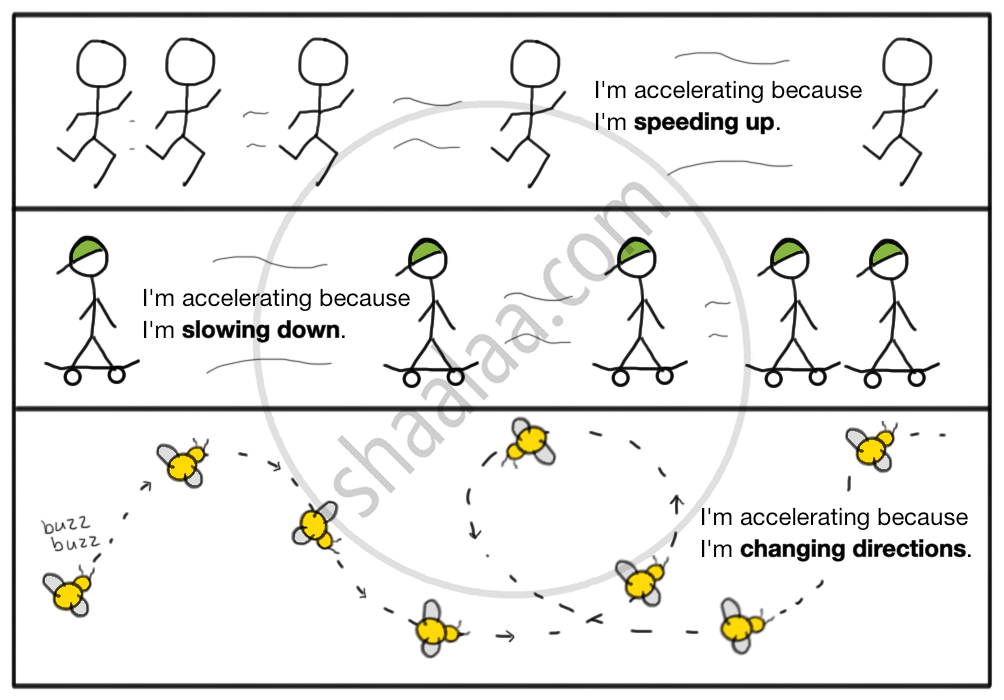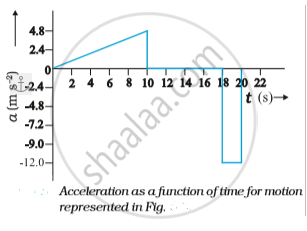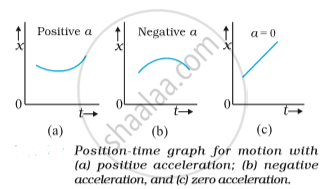# Acceleration (Average and Instantaneous)

## Acceleration

It is the rate of change of velocity with time. The only two ways to accelerate is by changing the speed or change in direction or change both. It is a vector quantity. If the velocity of the object increases with time, its acceleration increases. If the velocity of an object decreases with time, its acceleration is negative.

The motion is uniformly accelerated motion or non-uniformly accelerated, depending on how the velocity changes with time. It is uniform for a body if the velocity changes by equal amounts in equal intervals and if its velocity changes by unequal amounts, it is non-uniform.

Acceleration = Change in velocity/time taken
Its unit is m/s².Constant speed does not guarantee that acceleration is zero. For example, a body moving with constant speed in a circle changes its velocity every instant and hence its acceleration is not equal to zero.

Velocity is a quantity having both magnitude and direction, a change in velocity may involve either or both of these factors. Acceleration may result from a change in speed, a change in direction or changes in both. Like velocity, acceleration can also be positive, negative or zero.

Motion in Different Acceleration for Different Time Intervals
Let’s understand this through an example. Suppose, a particle started its motion from rest with an acceleration of 1m/s² for 2s and then continued it for next 1s changing to 2m/s². The distance travelled during this will be:

After 2s the velocity is, v = u + at = 2 m/s
Now, if this is the initial velocity for the second half of the motion, s_2=ut+1/2at²=3 m
Distance travelled in first half is: s_1 = 0+1/2at²= 2 m
Hence total distance travelled = s_1+s_2= 5 m

On a plot of velocity versus time, the average acceleration is the slope of the straight line connecting the points corresponding to (v_2, t_2) and (v_1, t_1). The average acceleration for velocity-time graph shown above for different time intervals 0 s- 10 s, 10 s – 18 s, and 18 s – 20 s are:
0s - 10s,  bar a=((24-0)ms^-1)/((10-0)s)=2.4  ms^-1
10s - 18s,  bar a=((24-24)ms^-1)/((18-10)s)=0  ms^-1
18s - 20s,  bar a=((0-24)ms^-1)/((20-18)s)=2.4  ms^-1Let us see how velocity-time graph looks like for some simple cases as shown below. The shows velocity time graph for motion with constant acceleration for the following cases:

(a) An object is moving in a positive direction with a positive acceleration, for example the motion of the car between t = 0 s and t = 10 s.
(b) An object is moving in positive direction with a negative acceleration, for example, motion of the car between t = 18 s and 20 s.
(c) An object is moving in negative direction with a negative acceleration, for example the motion of a car moving from O in negative x-direction with increasing speed.
(d) An object is moving in positive direction till time t_1, and then turns back with the same negative acceleration, for example the motion of a car from point O to point Q till time t_1 with decreasing speed and turning back and moving with the same negative acceleration.

Average Acceleration
It is the change in velocity divided by an elapsed time. For instance, if the velocity of a marble increases from 0 to 60 cm/s in 3 seconds, its average acceleration would be 20 cm/s². This means that the marble’s velocity will increase by 20 cm/s every second.

It is the rate of change of velocity with respect to displacement
Acceleration is a = (dv)/dt
∴ a = (dv)/(dx/v)
a = v((dv)/dx)

Freefall object experiences an acceleration of g= 9.8m/s² in a downward direction that is towards the center of the earth. In upward direction it is -g = -9.8m/s²

#### description

• Acceleration
• Uniform acceleration
• Non-uniform acceleration
• Average acceleration
• Instantaneous acceleration
If you would like to contribute notes or other learning material, please submit them using the button below.

### Shaalaa.com

Straight line motion Part 12 (Acceleration) [00:09:58]
S
0%